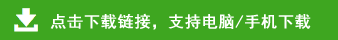# 2020-2021学年一年级数学上学期期中测试卷及答案

2020-2021学年一年级数学上学期期中测试卷及答案部分内容预览

一、算一算。(15分)
2+6=       7+3=　      4-4=
8+2=　 　3+5=         6-3=
8-2=        1+3=         0+8=
2+2=       10-7+3=    4+5+1=
2+5-4=    9-5-4=       8-1+2=
二、找规律,填一填。(3分)
三、看图填一填。(3分)
一共有(      )个图形,三角形是第(       )个,心形是第(        )个。
四、比一比,分一分。(15分)
1.在高的下面画“√”。(2分)
2.在最长的后面画“√”。(3分)
3. 在最重的小动物后面画“√”,在最轻的小动物后面画“○”。(6分)
4. 把每组中不同类的圈出来。(4分)
五、在○里填上“>”“<”或“=”。(16分)
7○9　        3○1
10○8　      4○4
6○3+3      1○4-1
6+3○6      5+2○3
六、按顺序填数。(10分)
七、看图列式。(18分)
八、解决问题。(20分)
1.现在有几只小鸟?(5分)
□○□=□
2. 原来草地上有7只小兔。(5分)
□○□=□
3. 车上原来有7人。(5分)
□○□○□=□
4. 小房子里原来有10只鸽子。(5分)
□○□○□=□